# Using VLOOKUP Tool for Microsoft ExcelIf any of the picture does not load properly in this article, i suggest visiting HERE  to learn vlookup.

## What is VLOOKUP Formula & how to use it?

I tell my excel school students that learning VLOOKUP formulas will change your basic approach towards data. You will suddenly feel that you have discovered a superman cape in your attic. It is that awesome.
What does VLOOKUP really do?
Imagine you have a list of data and you want answer a question like, "How many sales did Jimmy make?"
VLOOKUP is one of the formulas you can use in this situation. VLOOKUP searches a list for a value in left most column and returns corresponding value from adjacent columns.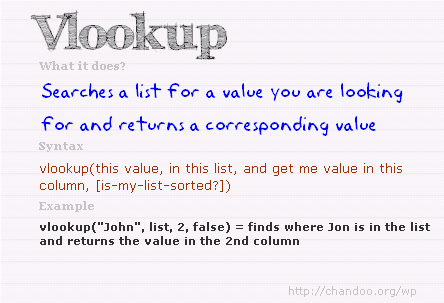Read more – What is VLOOKUP formula and how to use it?

## Introduction to VLOOKUP, MATCH & OFFSET formulas

VLOOKUP may not make you tall, rich and famous, but learning it can certainly give you wings. It makes you to connect two different tabular lists and saves a ton of time. In my opinion understanding VLOOKUP, OFFSET and MATCH worksheet formulas can transform you from normal excel user to a data processing beast.Read more – VLOOKUP, MATCH & OFFSET explained in plain English

## How to do wildcard searches with VLOOKUP?

Often we need our lookup formulas to go wild. Not in the sense of go-wild-and-chomp-a-few-kilo-bytes-of-data sense. But wild like wild cards. For eg. In the below data, we may not remember the full name of sales person, but we know that her name starts with jac. Now how do you get the sales amount for that person?
You can use wildcard characters * and ? with VLOOKUP & several other Excel formulas.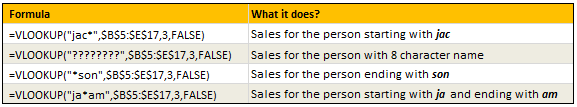Read more – Using wildcards with VLOOKUP formulas

## Making VLOOKUPS dynamic with data validation

Sometimes we don't know what we want. If this happens when I am in a bar, I usually order a cocktail. Just a mix of everything. The same will work in Excel too.
For eg. If you have lots of data, but the value you want to look up needs to change based on whims and fancies of your users, then you can resort to a cocktail. A mix of VLOOKUP with Drop down lists (Data validation).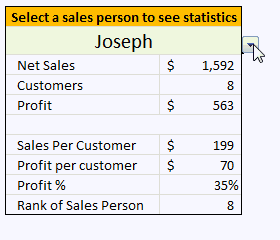Read more – Use data validation with VLOOKUP to lookup anything you want

## How to lookup values to the left?

There is no argument that VLOOKUP is a beautiful & useful formula. But it suffers from one nagging limitation. It cannot go left.
Let me explain, Imagine you have data like below. Now, if you want to find-out who is the sales person who made \$2,133 in sales, there is no way VLOOKUP can come to rescue. This is because, once you search a list using VLOOKUP, you can only return corresponding items from the column at right, not at left.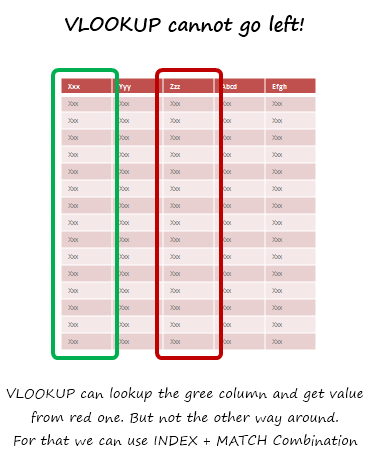Read more – How to use INDEX + MATCH combination to fetch values from left

## How to lookup based on multiple conditions?

Not always we want to lookup values based on one search parameter. For eg. Imagine you have data like below and you want to find how much sales Joseph made in January 2007 in North region for product "Fast car"? Read more to find how to solve this.
Read more – How to lookup based on multiple conditions?

## How to get values from multiple columns with VLOOKUP?

VLOOKUP is great for extracting information from a huge data table based on what you are looking for. But what if you need to extract more than one column of information? For eg. Lets say you have salesperson's name in left most column, and monthly sales figures in next columns, one for each month. Now, you want to find the total sales made by a given sales person. How do you go about it?
Read more – How to get values from multiple columns with VLOOKUP?

## Using VLOOKUP formula with tables

Excel Tables, a newly introduced feature in Excel 2007 is a very powerful way to manage & work with tabular data. I really like tables feature and use them often. If you are new to tables, read up Introduction to Excel Tables. In this short video, understand how to use tables with VLOOKUP formulas.
Watch the video – Using VLOOKUP formula with tables

## Doing 2 way lookups in Excel

So far we have seen what VLOOKUP formula is and how to put it to some nifty uses. Lets go one step further and learn how to do 2 Way Lookups.
What is a 2 Way Lookup?
Lookup is when you find a value in one column and get the corresponding element from other columns. 2 Way Lookup is when you lookup value at the interesection of a given row & column values.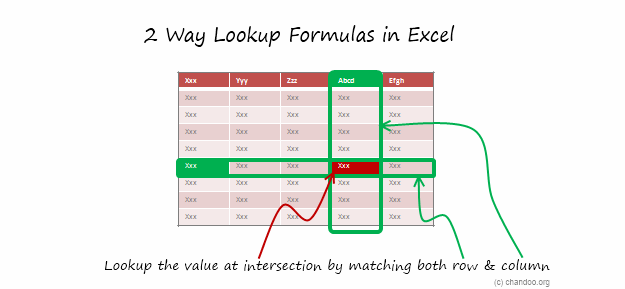Read more – 2 way lookup formula in Excel

## Getting 2nd matching value from a list using VLOOKUP

We know that VLOOKUP formula is useful to fetch the first matching item from a list. So what would you do if you need 2nd (or 3rd etc.) matching item from a list?
Read more – Getting 2nd matching value using VLOOKUP

## Range lookups in Excel

Here is a really tricky problem. Recently I was given a data set like this (shown below) and asked to find the position of lookup value in the list. The only glitch is that, instead of values, the lookup table contained lower and upper boundaries of the values. See the below illustration to understand the situation. In this case, how do you lookup?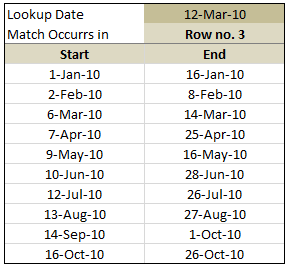Read more – Doing range lookups in Excel